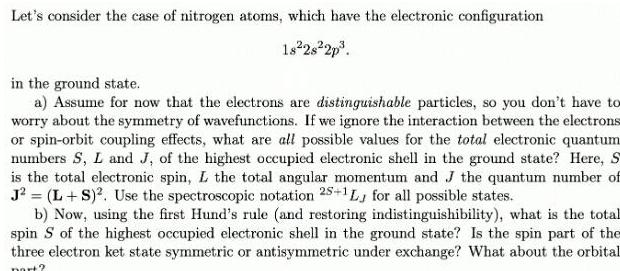Question:

# Let s consider the case of nitrogen atoms which have the

Last updated: 7/4/2023Let s consider the case of nitrogen atoms which have the electronic configuration 18 28 2p in the ground state a Assume for now that the electrons are distinguishable particles so you don t have to worry about the symmetry of wavefunctions If we ignore the interaction between the electrons or spin orbit coupling effects what are all possible values for the total electronic quantum numbers S L and J of the highest occupied electronic shell in the ground state Here S is the total electronic spin L the total angular momentum and J the quantum number of J L S Use the spectroscopic notation 25 1L for all possible states b Now using the first Hund s rule and restoring indistinguishibility what is the total spin S of the highest occupied electronic shell in the ground state Is the spin part of the three electron ket state symmetric or antisymmetric under exchange What about the orbital part